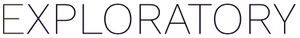# How to randomly populate values as a new column?

#1

Let’s say you have a data frame with 1000 rows, and you want to populate a column with values ranging from 1 to 12 (like month number).

You can use ‘runif’ function to randomly populate the values, which will be between 0 and 1, multiply by 12 to make the values between 0 and 12, then use ‘ceiling’ function to round up the values so that they’ll be integer values.

Here is the how the calculation look like.

``````ceiling(runif(n())*12)
``````

Here’s in Exploratory.

This ‘runif’ function is to populate random values according to ‘uniform’ distribution, which is basically the ‘same’ frequency for all the range.

If you want to use different distributions such as Normal distribution, then you can use ‘rnorm’ function instead.

``````ceiling(rnorm(n())*12)
``````